# iddata/plot

Plot input-output data

## Syntax

``plot(data)``
``plot(data,LineSpec)``
``plot(data1,...,dataN)``
``plot(data1,LineSpec1...,dataN,LineSpecN)``
``plot(axes_handle,___)``
``plot(___,plotoptions)``
``h = plot(___)``

## Description

example

````plot(data)` plots the input and output channels of an `iddata` object. The outputs are plotted on the top axes and the inputs on the bottom axes.For time-domain data, the input and output signals are plotted as a function of time. Depending on the `InterSample` property of the `iddata` object, the input signals are plotted as linearly interpolated curves or as staircase plots. For example, if `data.InterSample = 'zoh'`, the input is piecewise constant between sampling points, and it is then plotted accordingly.For frequency-domain data, the magnitude and phase of each input and output signal is plotted over the available frequency span.To plot a subselection of the data, use subreferencing:`plot(data(201:300))` plots the samples 201 to 300 in the dataset `data`.`plot(data(201:300,'Altitude',{'Angle_of_attack','Speed'}))` plots the chosen samples of output named `Altitude` and inputs named `Angle_of_attack` and `Speed`.`plot(data(:,[3 4],[3:7]))` plots all samples of output channel numbers 3 and 4 and input numbers 3 through 7.```
````plot(data,LineSpec)` specifies the color, line style and marker symbol for the dataset.```
````plot(data1,...,dataN)` plots multiple datasets. The number of plot axes are determined by the number of unique input and output names among all the datasets.```

example

````plot(data1,LineSpec1...,dataN,LineSpecN)` specifies the line style, marker type, and color for each dataset. You can mix `data,LineSpec` pairs with `data`. For example, `plot(data1,data2,LineSpec2,data3)`.```

example

````plot(axes_handle,___)` plots into the axes with handle `axes_handle` instead of into the current axes (`gca`). This syntax can include any of the input argument combinations in the previous syntaxes.```

example

````plot(___,plotoptions)` specifies the plot options. This syntax can include any of the input argument combinations in the previous syntaxes.```

example

````h = plot(___)` returns the handle to the plot. You can use this handle to customize the plot with `getoptions` and `setoptions`.```

## Input Arguments

collapse all

Input-output data, specified as an `iddata` object. The data can be time-domain or frequency-domain. It can be a single- or multi-channel data, or single- or multi-experiment data.

Line style, marker symbol, and color, specified as a character vector. `LineSpec` takes values such as `'b'`, `'b+:'`. For more information, see the `plot` reference page in the MATLAB® documentation.

Data Types: `char`

Axes handle, which is the reference to an axes object. Use the `gca` function to get the handle to the current axes, for example, ```axes_handle= gca```.

Plot options, specified as an option set created using `iddataPlotOptions`.

## Output Arguments

collapse all

Lineseries handle, returned as a scalar or vector. These are unique identifiers, which you can use to query and modify properties of a specific plot.

## Examples

collapse all

`load iddata1 z1;`

Plot the data.

`plot(z1)`The output is plotted on the top axes and the input on the bottom axes.

You can right-click the plot to explore its characteristics such as peak and mean values.

`load iddata1 z1`

Convert the data to frequency domain.

`zf = fft(z1);`

Plot the data.

`plot(zf);`Generate input data.

```u = idinput([100 1 20],'sine',[],[],[5 10 1]); u = iddata([],u,1,'per',100);```

Generate output data.

```sys = idtf(1,[1 2 1]); y = sim(sys,u);```

Plot only the input.

`plot(u)`Plot only the output.

`plot(y)`Plot the input and output together.

`plot(y,u)`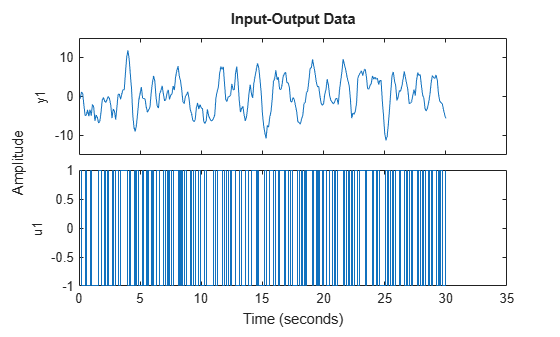Alternatively, you can use `plot(iddata(y,u))` .

Create a multi-experiment data set.

```load iddata1 z1 load iddata2 z2 zm = merge(z1,z2);```

Plot the data.

`plot(zm);`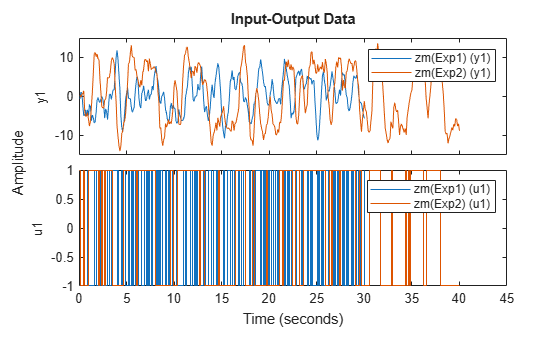For multi-experiment data, each experiment is treated as a separate data set. You can right-click the plots to view their characteristics.

```load iddata1 z1; load iddata2 z2;```

Specify the linestyle properties.

`plot(z1,'y:*',z2,'b')`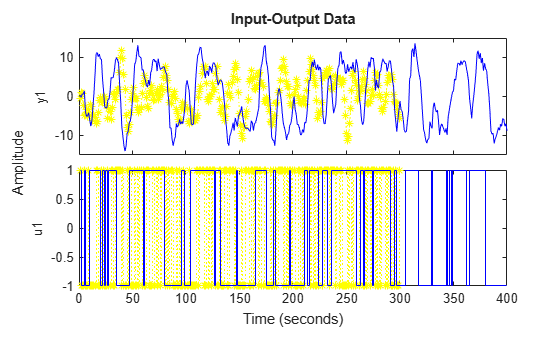Create a figure with two subplots and return the handle to each subplot axes, s(1) and s(2).

```figure; % new figure s(1) = subplot(1,2,1); % left subplot s(2) = subplot(1,2,2); % right subplot ``````load iddata1; load iddata2; ```

Create a data plot in each axes referring to the axes handles.

```plot(s(1),z1) plot(s(2),z2) ```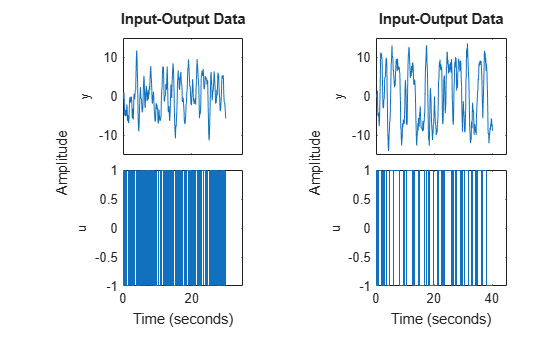Configure a time plot.

`opt = iddataPlotOptions('time');`

View the plot in minutes time units.

`opt.TimeUnits = 'minutes';`

Turn grid on.

`opt.Grid = 'on';`

Create plot with the options specified by opt.

```load iddata1 z1 plot(z1, opt);```Create a data plot and return the handle.

```load iddata1; h = plot(z1);```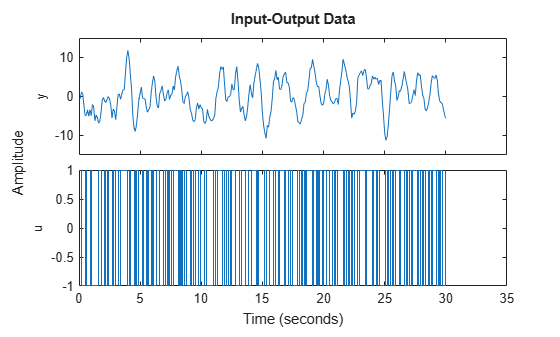Set the time unit on the plot.

`setoptions(h,'TimeUnits','minutes');`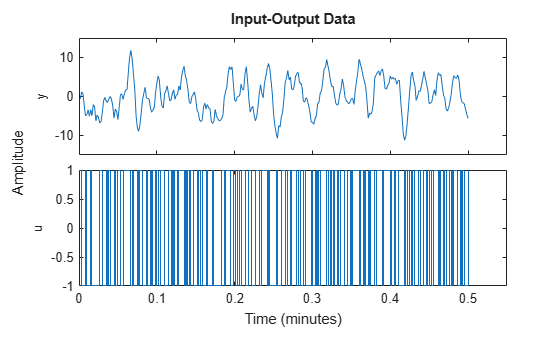Generate data with two inputs and one output.

`z = iddata(randn(100,1),rand(100,2));`

Configure a time plot.

`opt = iddataPlotOptions('time');`

Plot the data.

`h = plot(z,opt);`Change the orientation of the plots such that all inputs are plotted in one column, and all outputs are in a second column.

```opt.Orientation = 'two-column'; h = plot(z,opt);```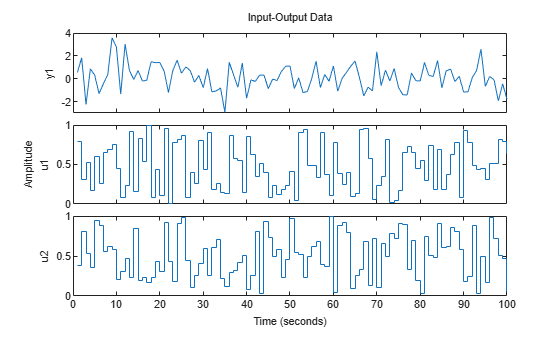Alternatively, use `setoptions`.

`setoptions(h,'Orientation','two-column')`

You can also change the orientation by right-clicking the plot and choosing `Orientation` in the context menu.

## Tips

• Right-clicking the plot opens the context menu where you can access the following options and plot controls:

• Datasets — View the datasets used in the plot.

• Characteristics — View data characteristics.

• Peak Value — Peak value of the data. Useful for transient data.

• Mean Value — Mean value of the data. Useful for steady-state data.

• Orientation — Choose orientation of the input and output plots.

• Output row and input row — (For datasets containing more than one input or output channel) Plot all outputs in one row and all inputs in a second row.

• Output column and input column — (For datasets containing more than one input or output channel) Plot all outputs in one column and all inputs in a second column.

• Single row — Plot all inputs and outputs in one row.

• Single column— Plot all inputs and outputs in one column.

• I/O Grouping — (For datasets containing more than one input or output channel) Group input and output channels on the plot.

• I/O Selector — (For datasets containing more than one input or output channel) Select a subset of the input and output channels to plot. By default, all input and output channels are plotted.

• Normalize — Normalize the y-scale of all data in the plot.

• Properties — Open the Property Editor dialog box, where you can customize plot attributes.